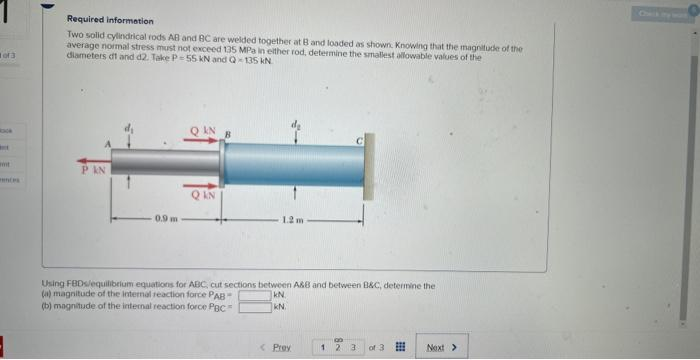Home / Expert Answers / Mechanical Engineering / required-information-two-solid-cylindirical-rods-mathrm-ab-and-mathrm-bc-are-welded-pa400

# (Solved): Required information Two solid cylindirical rods $$\mathrm{AB}$$ and $$\mathrm{BC}$$ are welded ...Required information Two solid cylindirical rods $$\mathrm{AB}$$ and $$\mathrm{BC}$$ are welded together at $$\mathrm{B}$$ and loaded as shown. Knowing that the magntiote of the average noimai stress must not enceed $$135 \mathrm{MPe}$$ in elther rod, determine the smallast allowable values of the diameters dt arid d2. Take $$P=55 \mathrm{kN}$$ and $$Q=135 \mathrm{kN}$$ Using FBDs/equilibelum equations for $$A B C$$, cut sections between $$A B B$$ and between $$B B C$$, deteririne the (i) magnitude of the internal reaction force $$P_{A B}=$$ (b) magnitude of the intemal reaction force $$\mathrm{PBC}_{\mathrm{B}}=$$ kN. $$\mathrm{kN}$$

We have an Answer from Expert